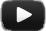0 (0 rates - 0 favorites)

# Soulblast - Turn This Place lyrics

You need to set me free
I count one, two, three
I need to take a break
I count one, two, three

I count one, two, three [2x]
Turn this place

Turn this place into a

Turn this place into a moshpit

Turn this place

Turn, turn this

Turn into a moshpit

You need to set me free

You need to set me free
I count one, two, three
I need to take a break
I count one, two, three
[2x]

One, two, three - go! (go [?x])
Turn this place

Turn this place in- (go!)

Turn this place into a

Into a moshpit

Turn this place into

One, two, three - go!

You need to set me free
I count one, two, three - one, two, one, go!
I need to take a break
I count one, two, three - turn this place

One, two, three - go!

Turn this place into a moshpit

Turn this place into a

Turn this place into a moshpit• Turn This Place

• Year
• 2023

• Genre

• SubmittedComment on this lyric
Register